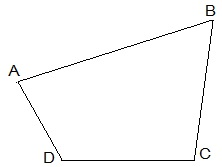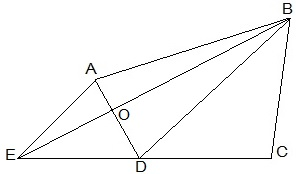# A villager Itwaari has a plot of land of the shape of a quadrilateral. The Gram Panchayat of the village decided to take over some portion of his plot from one of the corners to construct a Health Centre. Itwaari agrees to the above proposal with the condition that he should be given equal amount of land in lieu of his land adjoining his plot so as to form a triangular plot. Explain how this proposal will be implemented.Solution

Let ABCD be the plot of the land of the shape of a quadrilateralConstruction

Join the diagonal BD.

Draw AE parallel to BD.

Join BE, that intersected AD at O.

We get,

△BCE is the shape of the original field

△AOB is the area for constructing health centre.

△DEO is the land joined to the plot.

To prove:

ar(△DEO) = ar(△AOB)

Proof:

ΔDEB and ΔDAB lie on the same base BD, in-between the same parallels BD and AE.

Therefore,

Ar(ΔDEB) = ar(ΔDAB)

=> ar(ΔDEB) – ar(ΔDOB) = ar(ΔDAB) – ar(ΔDOB)

=> ar(ΔDEO) = ar(ΔAOB)(4)(5)